# 随笔

## 技术

2019-10-25   2.8k

# Machine_Learning-Week1

### 机器学习介绍

A computer program is said to learn from experience E with respect to some class of tasks T and performance measure P, if its performance at tasks in T, as measured by P, improves with experience E.

E：下很多场围棋的体验

T：某一局下棋的任务

P：DeepMind赢得比赛的可能性

#### 监督学习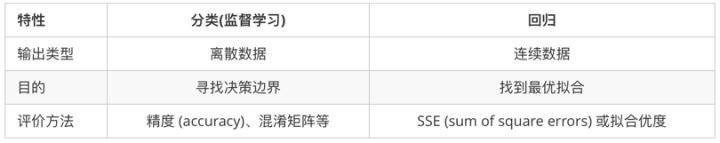### 单变量线性回归

#### 假设函数（Hypothesis Function）

$$\hat{y} = h_\theta(x) = \theta_0 + \theta_1 x$$

input x output y
0 4
1 7
2 7
3 8

#### 代价函数（Cost Function）

$$J(\theta_0, \theta_1) = \dfrac {1}{2m} \displaystyle \sum {i=1}^m \left ( \hat{y}{i}- y_{i} \right)^2 = \dfrac {1}{2m} \displaystyle \sum {i=1}^m \left (h\theta (x_{i}) - y_{i} \right)^2$$

$$\sum {i=1}^m \left (h\theta (x_{i}) - y_{i} \right)^2$$
i 为第 i 个数据，上式表示我通过拟合函数 hθ(x) 得到的第 i 个数据与真实的第 i 个数据的误差。总共有 m 个数据，那么我们就应该把 m 个数据的误差求和然后再求出平均误差，得到下面这个式子。
$$\dfrac {1}{2m} \displaystyle \sum {i=1}^m \left (h\theta (x_{i}) - y_{i} \right)^2$$

• 首先给了一个假设函数：

Hypothesis

$$\hat{y} = h_\theta(x) = \theta_0 + \theta_1 x$$

• 代价函数中的θ0和θ1是任意的，那么我们就需要一个函数来判断这个假设函数与真实数据之间的拟合度如何，为了描述拟合度，我们又提出了代价函数：
$$J(\theta_0, \theta_1) = \dfrac {1}{2m} \displaystyle \sum {i=1}^m \left ( \hat{y}{i}- y_{i} \right)^2 = \dfrac {1}{2m} \displaystyle \sum {i=1}^m \left (h\theta (x_{i}) - y_{i} \right)^2$$

• 我们的目标当然是代价函数的值越小越好，即用数学公式表示为：
$$\min_{\theta_1,\theta_2} J(\theta_1,\theta_2)$$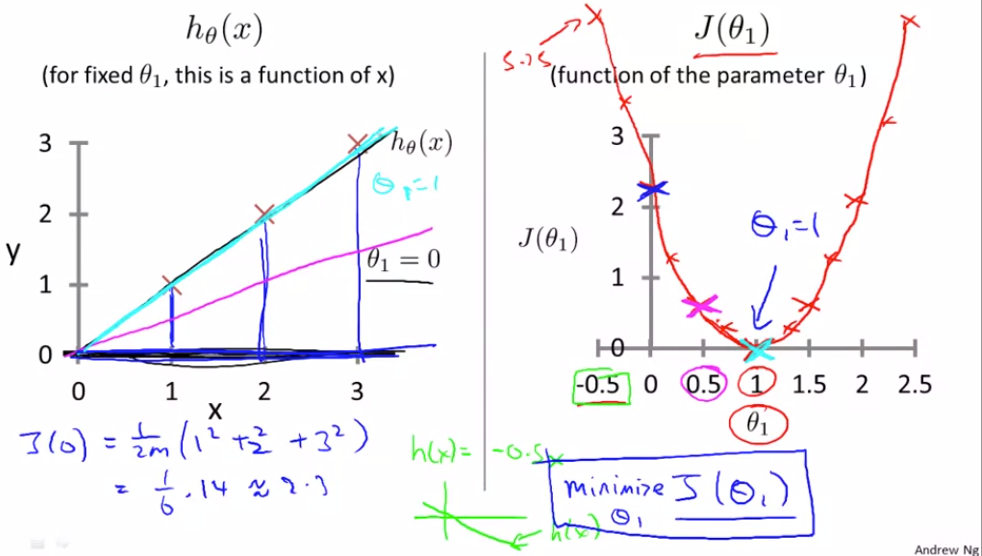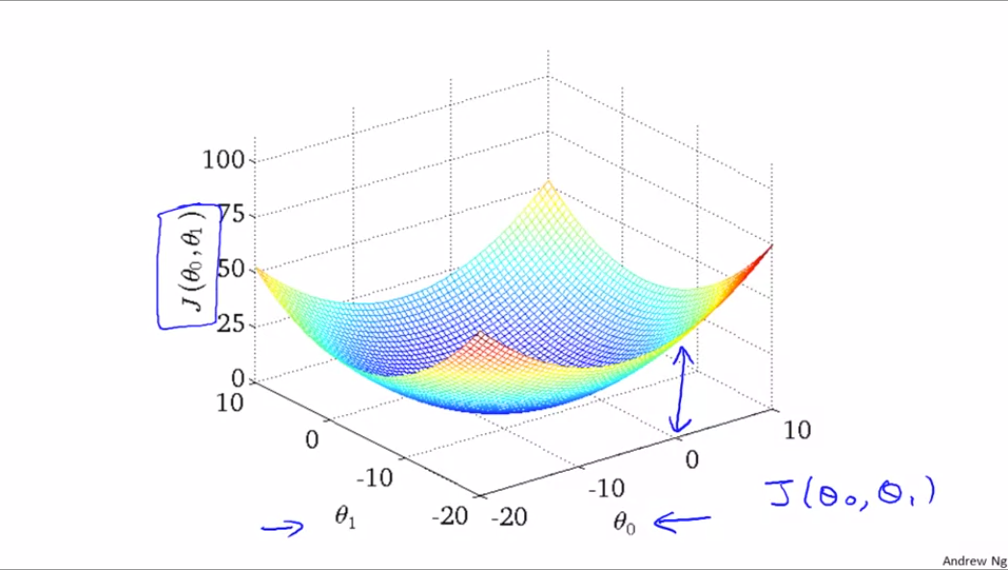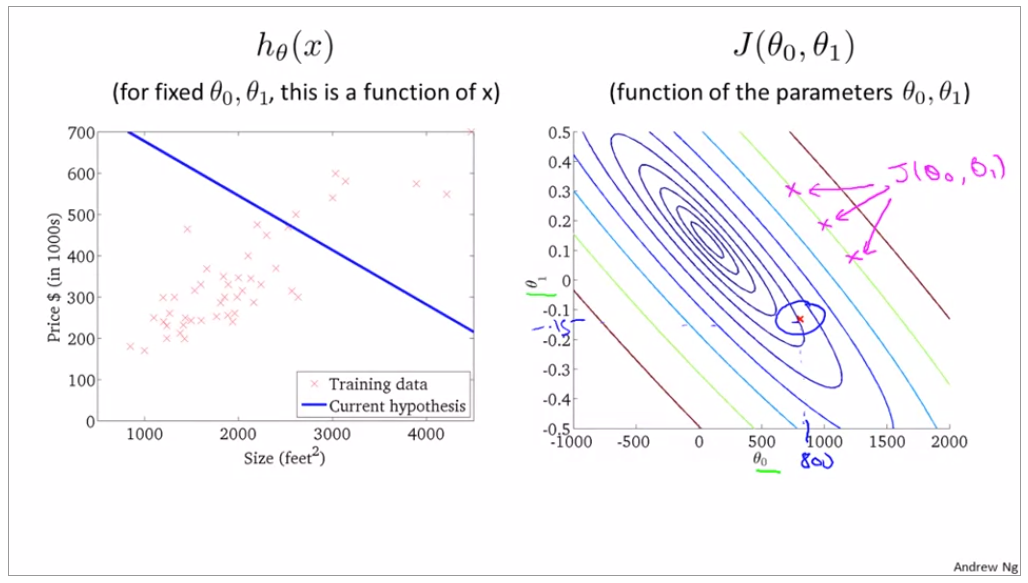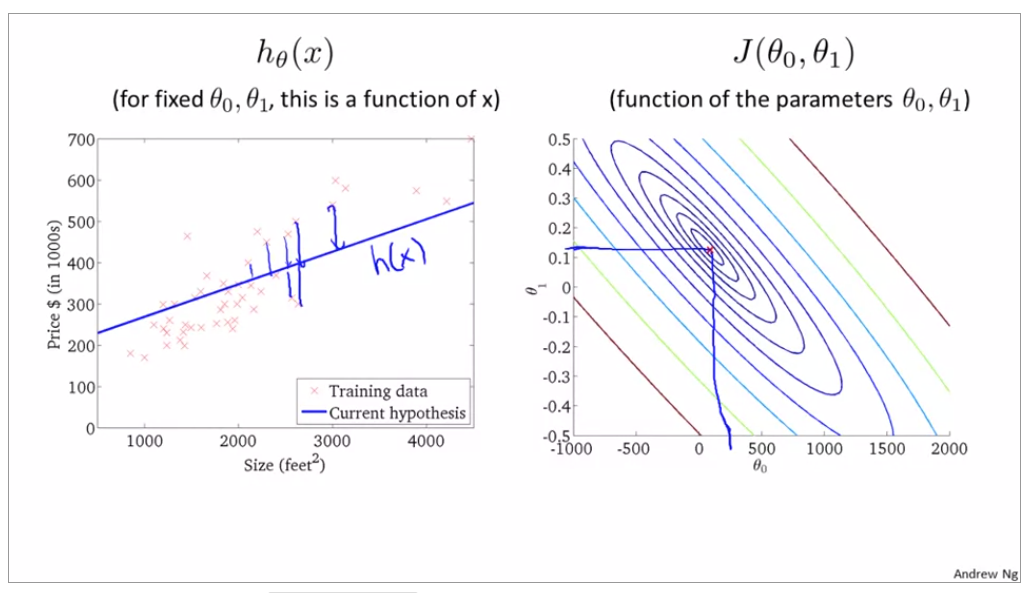**梯度下降（Gradient descent）**是一个用来求代价函数最小值的算法。梯度下降算法的思想就是首先先从一组参数值（θ0, θ1）开始，不断地去尝试各种（θ0, θ1），直到使得代价函数 J(θ0, θ1) 最小为止。以下图代价函数为例，从不同起始点开始，到达的局部最优位置不同，也就是局部最优解不同。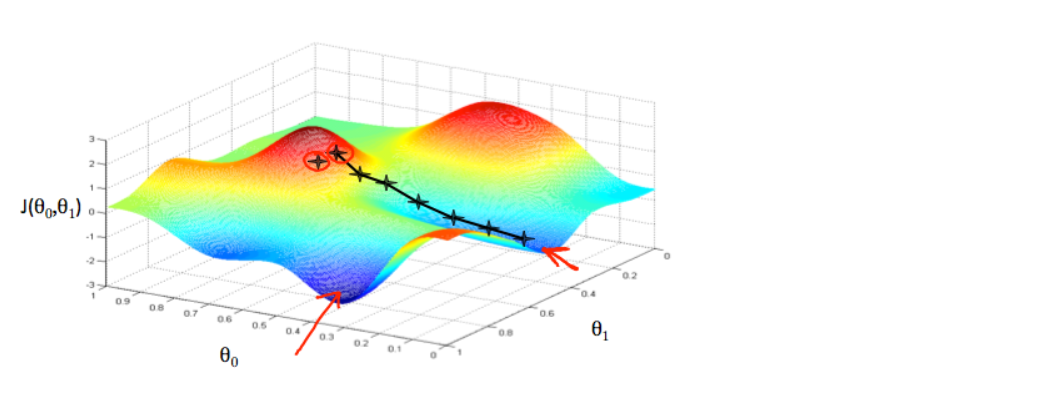repeat until convergence:
$$\theta_j := \theta_j - \alpha \frac{\partial}{\partial \theta_j} J(\theta_0, \theta_1)$$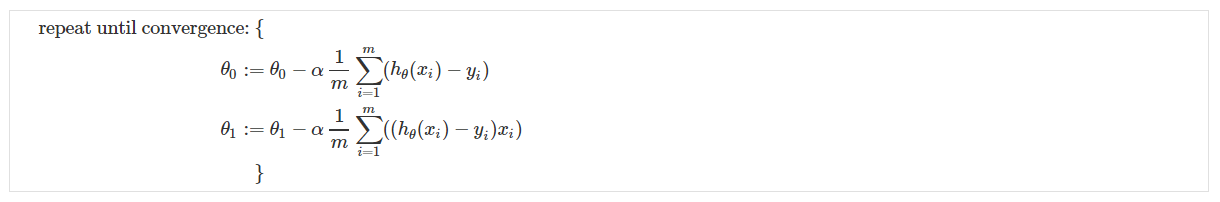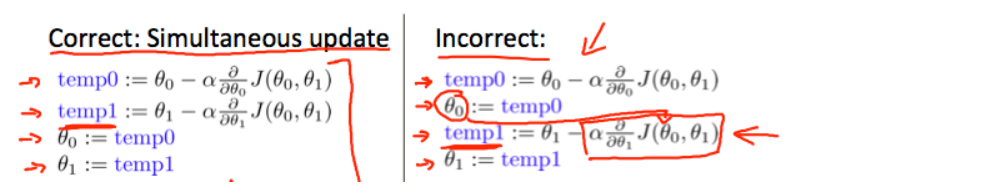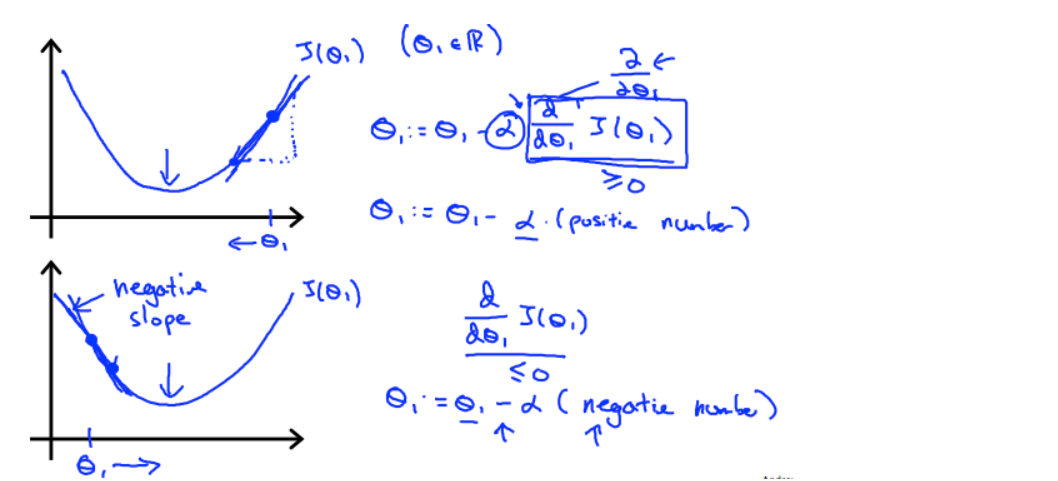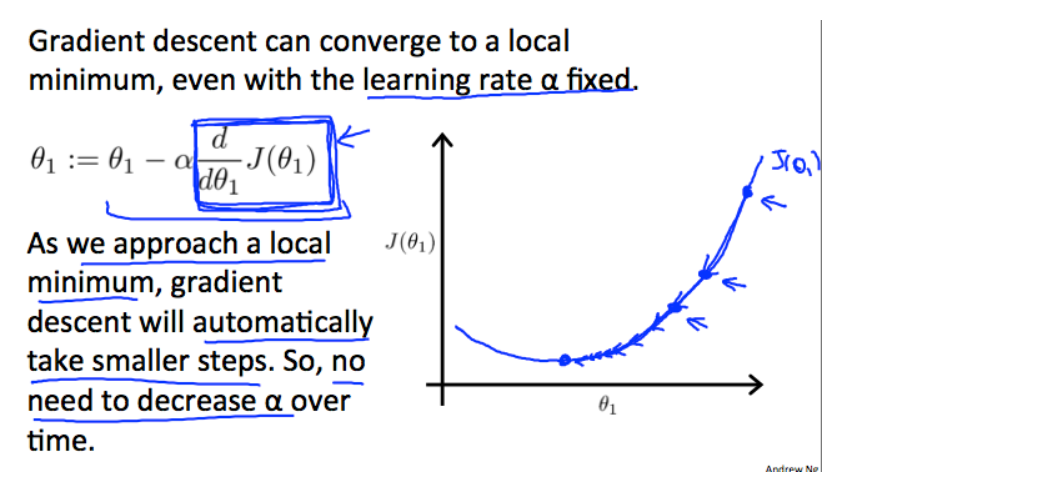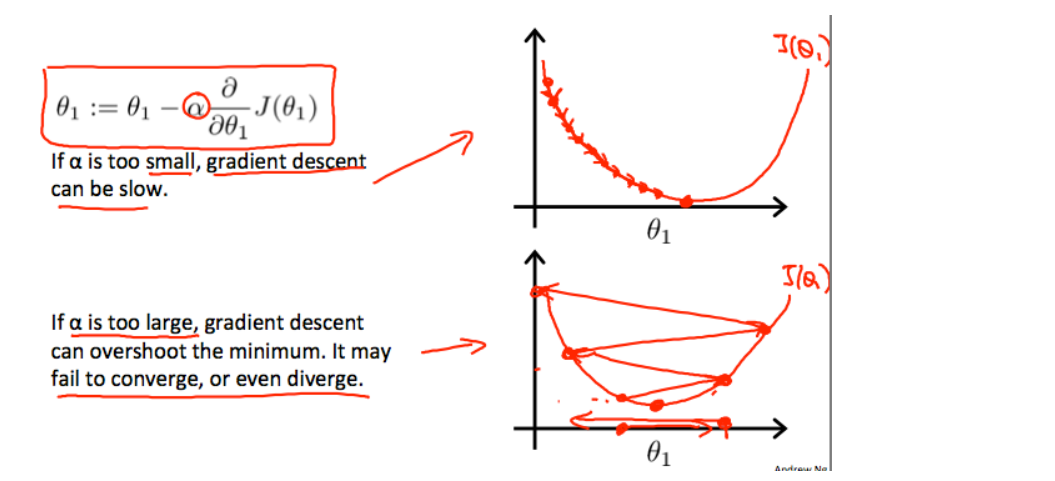### 线性回归的梯度下降算法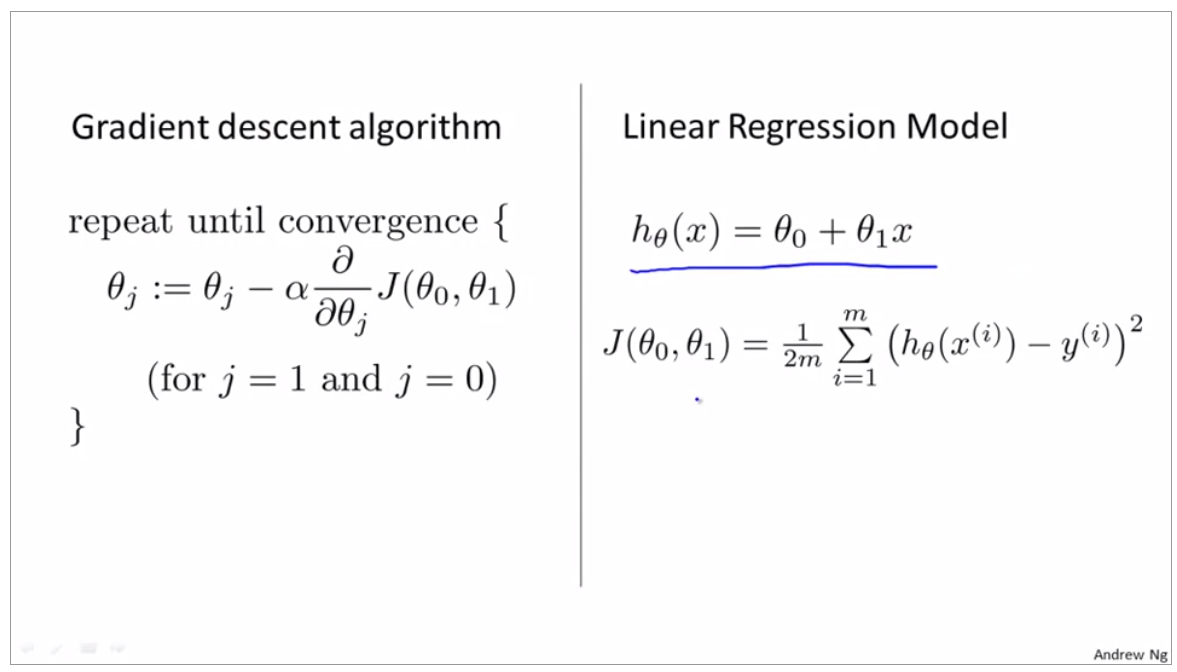$$\frac{\partial}{\partial \theta_0} J(\theta_0, \theta_1)=\dfrac {1}{m} \displaystyle \sum {i=1}^m \left (h\theta (x^{(i)}) - y^{(i)} \right)$$

$$\frac{\partial}{\partial \theta_1} J(\theta_0, \theta_1)=\dfrac {1}{m} \displaystyle \sum {i=1}^m \left ((h\theta (x^{(i)}) - y^{(i)} \right)x^{(i)}$$

\begin{align*} \text{repeat until convergence: } \lbrace & \newline \theta_0 := & \theta_0 - \alpha \frac{1}{m} \sum\limits_{i=1}^{m}(h_\theta(x_{i}) - y_{i}) \newline \theta_1 := & \theta_1 - \alpha \frac{1}{m} \sum\limits_{i=1}^{m}\left((h_\theta(x_{i}) - y_{i}) x_{i}\right) \newline \rbrace& \end{align*}